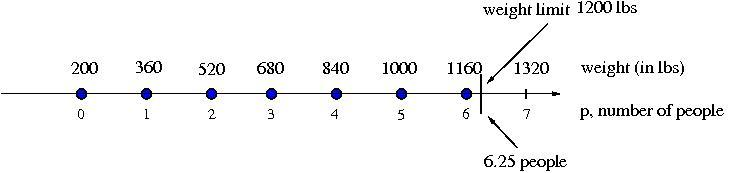Engage your students with effective distance learning resources. ACCESS RESOURCES>>

Alignments to Content Standards: 7.EE.B.4

Fishing Adventures rents small fishing boats to tourists for day-long fishing trips. Each boat can only carry 1200 pounds of people and gear for safety reasons. Assume the average weight of a person is 150 pounds. Each group will require 200 lbs of gear for the boat plus 10 lbs of gear for each person.

1. Create an inequality describing the restrictions on the number of people possible in a rented boat. Graph the solution set.
2. Several groups of people wish to rent a boat. Group 1 has 4 people. Group 2 has 5 people. Group 3 has 8 people. Which of the groups, if any, can safely rent a boat? What is the maximum number of people that may rent a boat?

IM Commentary

This task is the second in a series of three tasks that use inequalities in the same context at increasing complexity in 6th grade, 7th grade and in HS algebra. Students write and solve inequalities, and represent the solutions graphically. The progression of the content standards is 6.EE.8 to 7.EE.4 to A-REI.12.

This particular task could be used for instruction or assessment.

To see an annotated version of this and other Illustrative Mathematics tasks as well as other Common Core aligned resources, visit Achieve the Core.

Solution

1. Let $p$ be the number of people in a group that wishes to rent a boat. Then $150p$ represents the total weight of the people in the boat, in pounds. Also, $10p$ represents the weight of the gear that is needed for each person on the boat. So the total weight in the boat that is contributed solely by the people is $$150p+10p=160p$$ Because each group requires 200 pounds of gear regardless of how many people there are, we add this to the above amount. We also know that the total weight cannot exceed 1200 pounds. So we arrive at the following inequality: $$160p+200\leq 1200$$ A graph illustrating the solutions is shown below. We observe that our solutions are values of $p$, listed below the number line and shown by the blue dots, so that the corresponding weights $160 p+ 200$, listed above the line, are below the limit of $1200$ lbs.2. We can find out which of the groups, if any, can safely rent a boat by substituting the number of people in each group for $p$ in our inequality. We see that $$\mbox{For Group 1}: 160(4)+200=840\leq 1200$$ $$\mbox{For Group 2}: 160(5)+200=1000\leq1200$$ $$\mbox{For Group 3}: 160(8)+200=1480\not\leq1200$$ We find that both Group $1$ and Group $2$ can safely rent a boat, but that Group $3$ exceeds the weight limit, and so cannot rent a boat.

To find the maximum number of people that may rent a boat, we solve our inequality for $p$:

$$160p+200\leq1200$$ $$160p\leq1000$$ $$p\leq6.25$$

As we cannot have .25 person, we see that 6 is the largest number of people that may rent a boat at once. This also matches our graph; since only integer values of $p$ make sense, 6 is the largest value of $p$ whose corresponding weight value lies below the limit of $1200$ lbs.